## The RKT Trick: A 2-Generator Approach

RKT, formerly written out as <RK, T->, is a 2-generator approach to solving cell-turning puzzles.

### The notation

On the four-dimensional Rubik’s cube, turns are described using two-colour (2c) pieces. We label the cells U, R, L, F, B, D, T, and K, where T stands for top, and K stands for kata. Below is an image of the 3x3x3x3: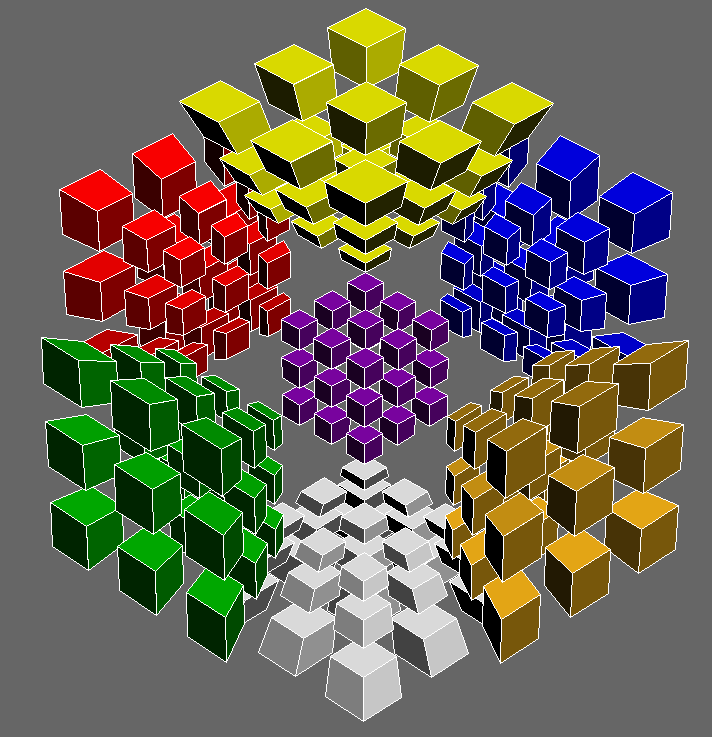The yellow cell is U, the green cell is F, the orange cell is R, the red cell is L, the blue cell is B, the white cell is D, and the violet cell is T. Notice that the cyan K cell is not rendered due to projection. If it were rendered, the rest of the puzzle would be hidden by that cell alone. Since the puzzle is fully functional, 4D rotations allow solvers to access the cyan cell during the solve.

Now, given two adjacent cells X and Y, XY means to turn the X cell clockwise 90 degrees along the axis of the 2c piece between X and Y. Thus, RK describes the following turn: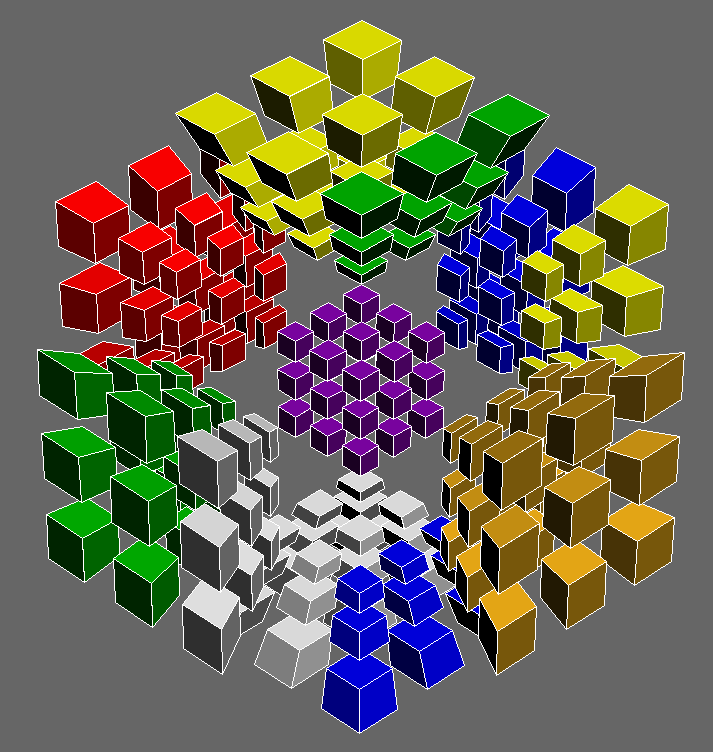T- then stands for the set of all turns of the form TX, where X is a cell adjacent to T. Similarly, -T stands for the set of all turns of the form XT.

Thus, RKT essentially stands for the use of RK and T- turns.

### The goal of RKT

RKT allows the solver to move around pieces on the T cell without disturbing other cells. That is, the T cell essentially becomes a 3D face-turning puzzle. Thus, for the 4D Rubik’s cube, RKT allows the solver to treat the T cell like a 3D Rubik’s cube.

Consider a clockwise A-perm. On the 3D Rubik’s cube, an algorithm for that case is
x' R' D R' U2 R D' R' U2 R2 x. We want to transform this algorithm into its RKT equivalent. However, we can only use RK and T- turns. The trick is to first consider how the A-perm would be executed if only the move R, along with any rotation, could be used. x' R' D R' U2 R D' R' U2 R2 x then becomes x' R' z' R z R' z R2 z' R z' R' z R' z R2 z' R2 x.

Now, to translate this into RKT, replace R with RK, and z with TF, since z is equivalent to rotating the entire cube in the same direction as an F turn. The x rotations can technically be replaced with TRs, too, though in this case, they can also be left unchanged. Thus, the RKT A-perm is x' RK' TF' RK TF RK' TF RK2 TF' RK TF' RK' TF RK' TF RK2 TF' RK2 x.

A picture of executing the regular A-perm on a 4D Rubik’s cube is as follows, where R=RK, D=DK, and U=UK: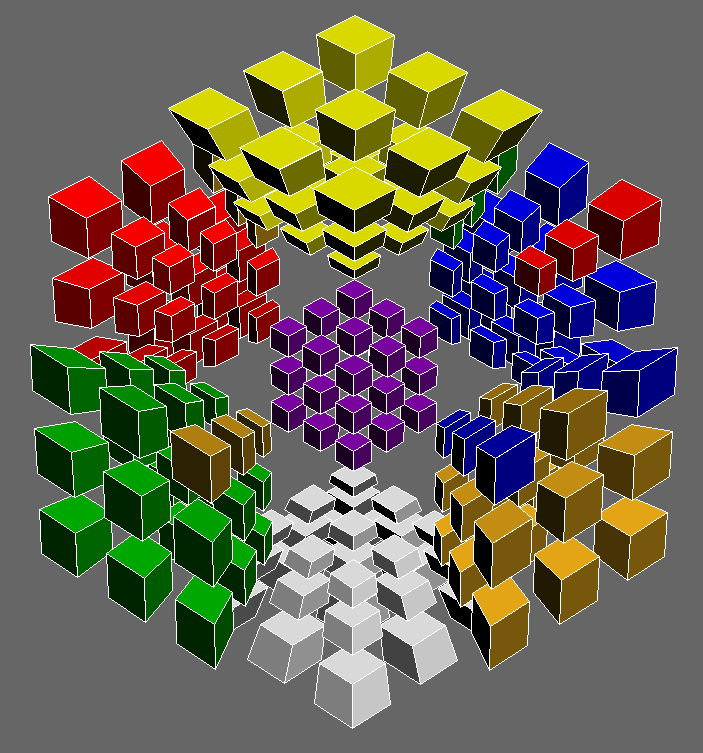A picture of executing the RKT A-perm on a 4D Rubik’s cube is as follows: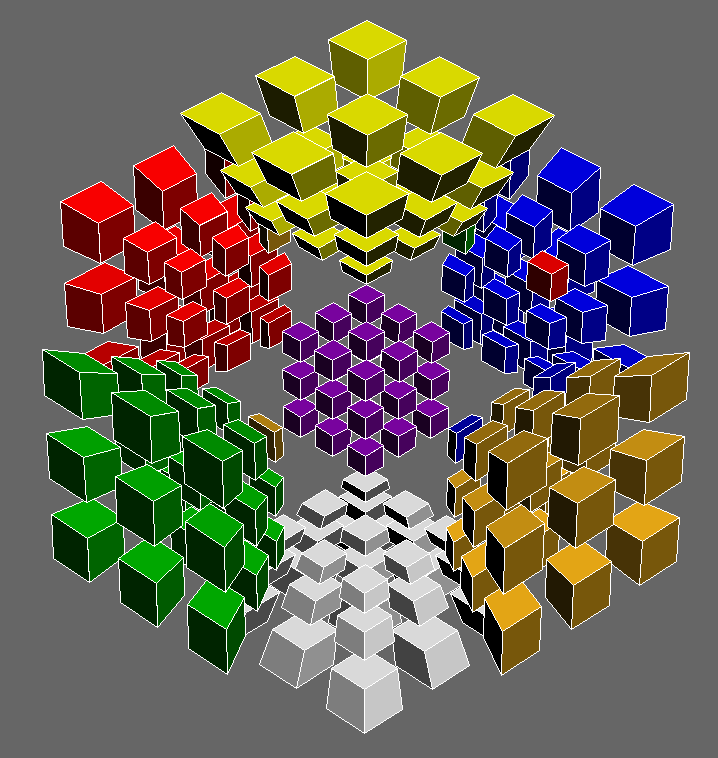We see that RKT has only modified the T-cell pieces, as desired. We can also note that RKT is especially good for algorithms that only need to affect 4c pieces, such as this one.

### Generalizing RKT by varying face turns

There is no requirement that the T cell is the one being treated as a 3D Rubik’s cube. In fact, by using TU and D- instead of RK and T-, we can solve 3c/4c pairs using “RKT” versions of F2L inserts. For example, consider the insertion of the 3c/4c pair on the DTR edge using an “RKT” U R U’ R’, where instead of RK, we use TU, and instead of T-, we use D-.

Before: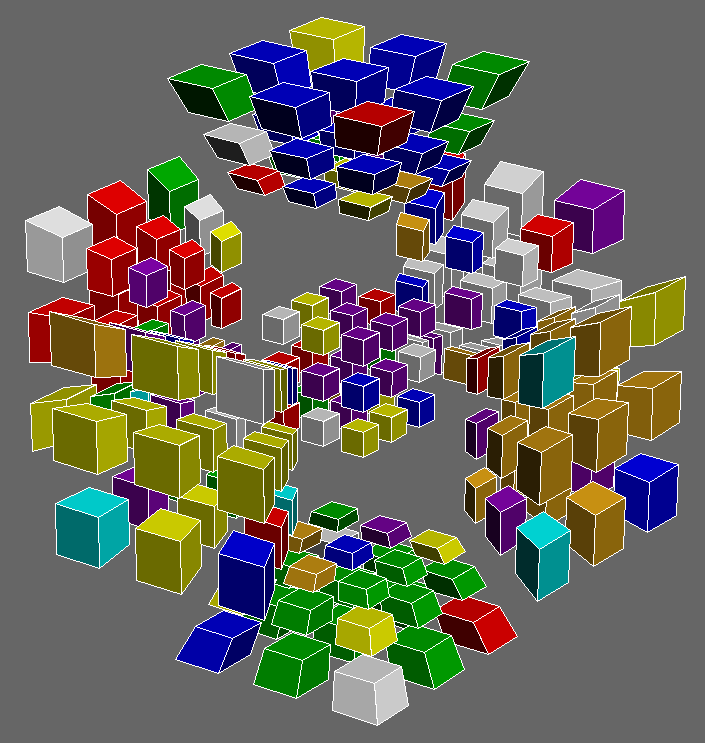After: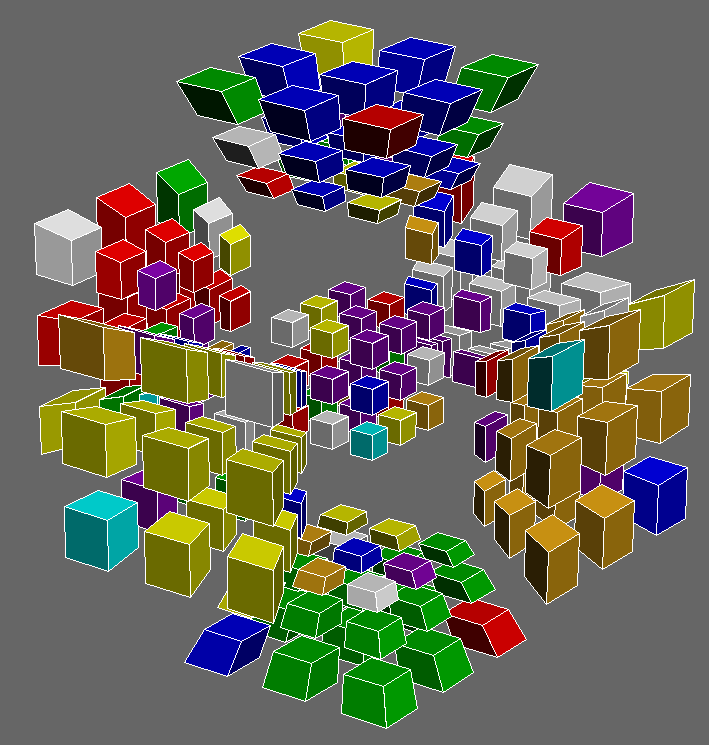Notice that even though TU and D- are the turns being used, the algorithm is still considered an “RKT” version of the U R U’ R’. Thus, RKT is not just a set of turns, but a general strategy to treat one cell as though it were its own 3D puzzle.

### Generalizing RKT to higher dimensions

RKT can be generalized to higher dimensions, but it is not recommended due to an exponential movecount increase, as well as being harder to manage.

For example, we can generalize RKT to the 5-dimensional 3x3x3x3x3, and treat the inner 3x3x3x1x1 as a 3x3x3 Rubik’s cube.

However, instead of just RK and T-, we now have to supply more moves in order to isolate and adjust the 3x3x3x3x1 T hypercell, which in turn isolates and adjusts its inner 3x3x3x1. Thus, the number of moves required to execute the equivalent 3-dimensional algorithm using RKT grows exponentially as the number of dimensions increase.

Go back to Articles